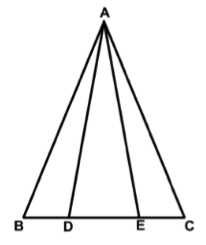### Prove: Triangle ABD is congruent to Triangle ACEClick or touch to draw on the diagram
Line BDEC, AB is congruent to AC, BD is congruent to CE
Triangle ABC is an isosceles triangle
If two sides of a triangle are equal, the angles opposite them are equal
Given
SAS (Side-Angle-Side)
Angle B is congruent to Angle C
Triangle ABD is congruent to Triangle ACE
Definition